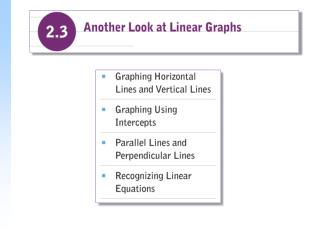# Lines with Zero Slope and Undefined Slope - PowerPoint PPT PresentationDownload PresentationLines with Zero Slope and Undefined Slope

Lines with Zero Slope and Undefined Slope
Download Presentation## Lines with Zero Slope and Undefined Slope

- - - - - - - - - - - - - - - - - - - - - - - - - - - E N D - - - - - - - - - - - - - - - - - - - - - - - - - - -
##### Presentation Transcript

1. Choose any number for x y must always be 2 Lines with Zero Slope and Undefined Slope Example Use slope-intercept form to graph f (x) = 2 Solution Writing in slope-intercept form: f (x) = 0 • x + 2. No matter what number we choose for x, we find that y must equal 2. f (x) = 2

2. Example continued y = f (x) = 2 When we plot the ordered pairs (0, 2), (4, 2) and (4, 2) and connect the points, we obtain a horizontal line. Any ordered pair of the form (x, 2) is a solution, so the line is parallel to the x-axis with y-intercept (0, 2).

3. x must be 2 Any number can be used for y Example Graph x = 2 Solution We regard the equation x = 2 as x + 0 • y = 2. We make up a table with all 2 in the x-column. x = 2

4. Example continued x = 2 When we plot the ordered pairs (2, 4), (2, 1), and (2, 4) and connect them, we obtain a vertical line. Any ordered pair of the form (2, y) is a solution. The line is parallel to the y-axis with x-intercept (2, 0).

5. Example • Find the slope of each given line. • a. 4y+ 3 = 15 b. 5x = 20 • Solution • a. 4y + 3 = 15 (Solve for y) • 4y = 12 • y = 3 • Horizontal line, the slope of the line is 0. • b. 5x = 20 • x = 4 • Vertical line, the slope is undefined.

6. x-intercepts (-1, 0) (3, 0) y-intercept (0, -3) Graphing Using Intercepts • The point at which the graph crosses the x-axis is called the x-intercept. The y-coordinate of a x-intercept is always 0, (a, 0) where a is a constant. • The point at which the graph crosses the y-axis is called the y-intercept. The x-coordinate of a y-intercept is always 0, (0, b) where b is a constant.

7. Solution To find the y-intercept we let x = 0 and solve for y. 5(0) + 2y = 10 2y = 10 y = 5 (0, 5) To find the x-intercept we let y = 0 and solve for x. 5x + 2(0) = 10 5x = 10 x = 2 (2, 0) y-intercept (0, 5) x-intercept (2, 0) 5x + 2y = 10 Example Graph 5x + 2y = 10 using intercepts.

8. Press Press Press Go to your y-editor and enter the equation. Select menu 1 Enter 0 So, the y-intercept is (0, -4).

9. Press Press Press Press Select 2 from the menu Enter 8 So, the x-intercept is (6, 0). Enter 3

10. Press Press Press

11. Parallel and Perpendicular Lines • Two lines are parallel if they lie in the same plane and do not intersect no matter how far they are extended. If two lines are vertical, they are parallel.

12. Example Determine whether the graphs of and 3x 2y = 5 are parallel. • Solution When two lines have the same slope but different y-intercepts they are parallel. • The line has slope 3/2 and y-intercept (0, 3). • Rewrite 3x 2y = 5 in slope-intercept form: • 3x 2y = 5 • 2y = 3x  5 • The slope is 3/2 and the y-intercept is (5/2, 0). • Both lines have slope 3/2 and different y-intercepts, the graphs are parallel.

13. Example Determine whether the graphs of 3x 2y = 1 and are perpendicular. • Solution • First, we find the slope of each line. • The slope is – 2/3. • Rewrite the other line in slope-intercept form. The slope of the line is 3/2. The lines are perpendicular if the product of their slopes is 1. The lines are perpendicular.

14. The slope is Example • Write a slope-intercept equation for the line whose graph is described. • a) Parallel to the graph of 4x 5y = 3, with y-intercept (0, 5). • b) Perpendicular to the graph of 4x 5y = 3, • with y-intercept (0, 5). • Solution (a) • We begin by determining the slope of the line. A line parallel to the graph of 4x 5y = 3 has a slope of Since the y-intercept is (0, 5), the slope-intercept equation is

15. continued • b) A line perpendicular to the graph of 4x 5y = 3 has a slope that is the negative reciprocal of • Since the y-intercept is (0, 5), the slope-intercept equation is

16. Press Press Select 5 from the menu They don’t appear to be Perpendicular. That’s better!

17. Recognizing Linear Equations • A linear equation may appear in different forms, but all linear equations can be written in standard form Ax + By = C.

18. Example • Determine whether each of the following equations is linear: • a) y = 4x + 2 b) y = x2 + 3 • Solution Attempt to write each equation in standard form. • a) y = 4x + 2 • 4x + y = 2 Adding 4x to both sides b) y = x2 + 3 x2 + y = 3 Adding x2 to both sides The equation is not linear since it has an x2 term.